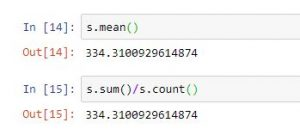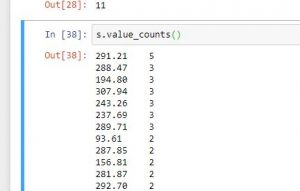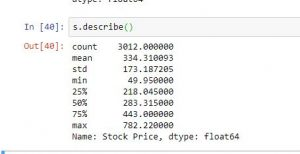# Python | Math operations for Data analysis

Python is a great language for doing data analysis, primarily because of the fantastic ecosystem of data-centric Python packages. Pandas is one of those packages, and makes importing and analyzing data much easier.

There are some important math operations that can be performed on a pandas series to simplify data analysis using Python and save a lot of time.

```s=read_csv("stock.csv", squeeze=True)
#reading csv file and making seires
```
Function Use
s.sum() Returns sum of all values in the series
s.mean() Returns mean of all values in series. Equals to s.sum()/s.count()s.std() Returns standard deviation of all values
s.min() or s.max() Return min and max values from series
s.idxmin() or s.idxmax() Returns index of min or max value in series
s.median() Returns median of all value
s.mode() Returns mode of the series
s.value_counts() Returns series with frequency of each values.describe() Returns a series with information like mean, mode etc depending on dtype of data passedCode #1:

 `# import pandas for reading csv file ` `import` `pandas as pd ` ` `  `#reading csv file ` `s ``=` `pd.read_csv(``"stock.csv"``, squeeze ``=` `True``) ` ` `  `#using count function ` `print``(s.count()) ` ` `  `#using sum function ` `print``(s.``sum``()) ` ` `  `#using mean function ` `print``(s.mean()) ` ` `  `#calculatin average ` `print``(s.``sum``()``/``s.count()) ` ` `  `#using std function ` `print``(s.std()) ` ` `  `#using min function ` `print``(s.``min``()) ` ` `  `#using max function ` `print``(s.``max``()) ` ` `  `#using count function ` `print``(s.median()) ` ` `  `#using mode function ` `print``(s.mode()) `

Output:

```3012
1006942.0
334.3100929614874
334.3100929614874
173.18720477113115
49.95
782.22
283.315
0    291.21```

Code #2:

 `# import pandas for reading csv file ` `import` `pandas as pd ` ` `  `#reading csv file ` `s ``=` `pd.read_csv(``"stock.csv"``, squeeze ``=` `True``) ` ` `  `#using describe function ` `print``(s.describe()) ` ` `  `#using count function ` `print``(s.idxmax()) ` ` `  `#using idxmin function ` `print``(s.idxmin()) ` ` `  `#count of elements having value 3 ` `print``(s.value_counts().head(``3``)) `

Output:

```dtype: float64
count    3012.000000
mean      334.310093
std       173.187205
min        49.950000
25%       218.045000
50%       283.315000
75%       443.000000
max       782.220000
Name: Stock Price, dtype: float64

3011
11
291.21    5
288.47    3
194.80    3
Name: Stock Price, dtype: int64
```

Unexpected Outputs and Restrictions:

1. .sum(), .mean(), .mode(), .median() and other such mathematical operations are not applicable on string or any other data type than numeric value.
2. .sum() on a string series would give an unexpected output and return a string by concatenating every string.

My Personal Notes arrow_drop_upCheck out this Author's contributed articles.

If you like GeeksforGeeks and would like to contribute, you can also write an article using contribute.geeksforgeeks.org or mail your article to contribute@geeksforgeeks.org. See your article appearing on the GeeksforGeeks main page and help other Geeks.

Please Improve this article if you find anything incorrect by clicking on the "Improve Article" button below.

Article Tags :

2

Please write to us at contribute@geeksforgeeks.org to report any issue with the above content.